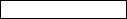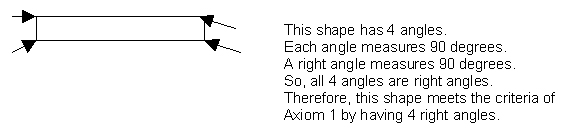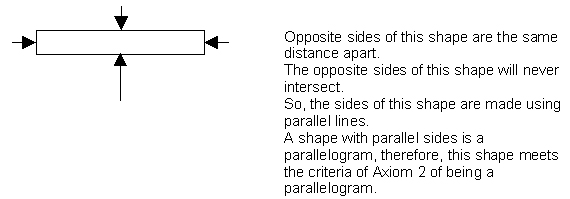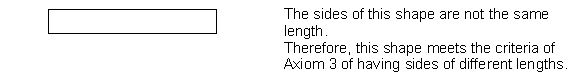Understanding Geometry Proofs

# Understanding Geometry Proofs

As geometric concepts develop and progress along a continuum of difficulty, it becomes more and more important to take information learned and apply it in a new and exciting ways. This process of applying previously known knowledge through deductive reasoning is necessary to further developing not only an understanding of geometry, but also in developing the ability to think in a deductive manner.
Want to learn more? Take an online course in Geometry.

Beginning to draw conclusions based on the information provided is the first step in understanding geometrical proofs. In the simplest terms, a proof is the process of drawing as many true conclusions about the information provided as possible to gain the narrowest viewpoint about what is presented. The proof is the written description of the process used to reach the conclusion. There is never simply one correct answer for a proof, as with any thinking process there are many different ways problems can be solved.

Proofs in geometry allow one to logically argue certain aspects about objects using geometric terms. It requires a step-by-step process based on known information about the shape or object. Proofs can be used to show objects are parallel, congruent or many other aspects. They could also be used to disprove the same concepts. Developing and creating proofs is typically based on very little information and requires the ability to think in a deductive manner.

In proofs, the true statements which are drawn from are considered theorems. The information you know about shapes (the definition which is known to be true) are considered axioms or postulates. In a true geometric proof, these axioms are used to develop theorems. The process used to develop a theorem is considered the proof. Geometric proofs are a combination of known definitions, postulates, axioms and theorems. Each piece is compiled and built upon the other in a step-by-step manner to arrive at one conclusion.

Definitions are usually the foundation of a geometric proof. Understanding the meaning of specific terms or objects is the beginning stage of being able to arrive at one conclusion. For instance, understanding that a four sided shape with all equal sides is known as a square is understanding the definition of a square. There are undefined terms in geometry. The undefined terms are those in which you can only arrive at a definition by using the word itself in the definition. Some examples of some undefined terms include: points, lines, and planes. Undefined terms help to provide a basis and understanding of future terms. They are important in having a complete understanding of the principles of geometry.

As discussed in the previous lesson, axioms and postulates are generally accepted facts about objects or numbers. There is no proof, but there is also no example which disproves them either. Until someone finds a nonexample, they are accepted as being true. Postulates generally refer to concepts of geometry while axioms, as previously discussed, pertain to real numbers. The reason there is no proof, is often, that it is impossible to prove. Consider the concept of a parallel line. It cannot be proven as no one can draw a line that goes on forever, as forever never ends. Though they cannot be proven, both postulates and axioms can be utilized in the process of completing a geometric proof.

Theorems, statements which can be proven, are often then used in later proofs. In other words, once a statement has been proven true, it is not necessary to prove each and every time it is to be used that it is true. It can be accepted that the theorem is true and can then be used to prove other geometric concepts. Geometric proofs are the typical end result and ultimate goal of geometric thinking.

Finally, as a last step it is imperative that one be familiar with the concepts of logic in order to solve a geometric proof. Logical statements are always declarative. They tell information, and do not ask questions. Logical statements can be conditional such as the if-then statements discussed in a previous lesson, but they do not have to have conditions. They can also be all encompassing such as the all statements discussed in the previous lesson. All logic statements in addition to be declarative are also definitively true or false. In other words, it cannot be argued. The application of logic is essential to the successful completion of a geometric proof.

For example:

Axiom 1 = Rectangles have 4 right angles.

Axiom 2 = Rectangles are parallelograms.

Axiom 3 = The sides of a rectangle do not have to be the same length.

Prove this shapeis a rectangle.

To complete this example, all three axioms must be used to show that the shape drawn meets all of the provided criteria and can therefore be concluded to be a rectangle. Remembering that the proof is the written process, one possible proof for might be:Therefore, as this shape meets all three axioms, it can be concluded that this shape is a rectangle.

Proofs begin very simple, but become more complex as concepts become more complicated and developed. Entire courses are built around understanding and writing proofs for geometric concepts. The process is often difficult and can be frustrating. Starting with the most basic truths and working slowly and methodically will help in applying the skills of deductive reasoning to geometric concepts.

One of the most difficult concepts in completing geometric proofs is the idea of where to begin. Often, it is through a process of trial and error which one must complete that geometric proofs are completed. Through the continued development and practice one can begin to arrive at a more consistent process for completing geometric proofs. Often, it begins by ruling out what information is not needed to be considered. Then applying the information which is known along with what definitions, axioms and postulates are also known or available. It is sometimes difficult to determine what is relevant and what is not relevant when considering the limited information typically given at the beginning of a proof. In some cases, it is through the trial and error process that proofs are solved. However, there are more complex proofs which can only be solved if specific steps are taken in exactly the right order. Patience, as stated before is often the key to being successful with deductive reasoning and solving any geometric proof.

Explore